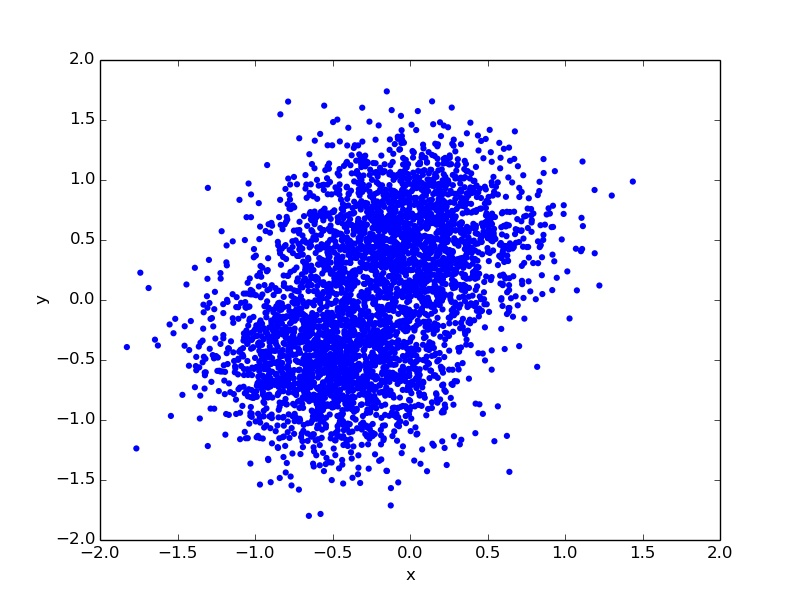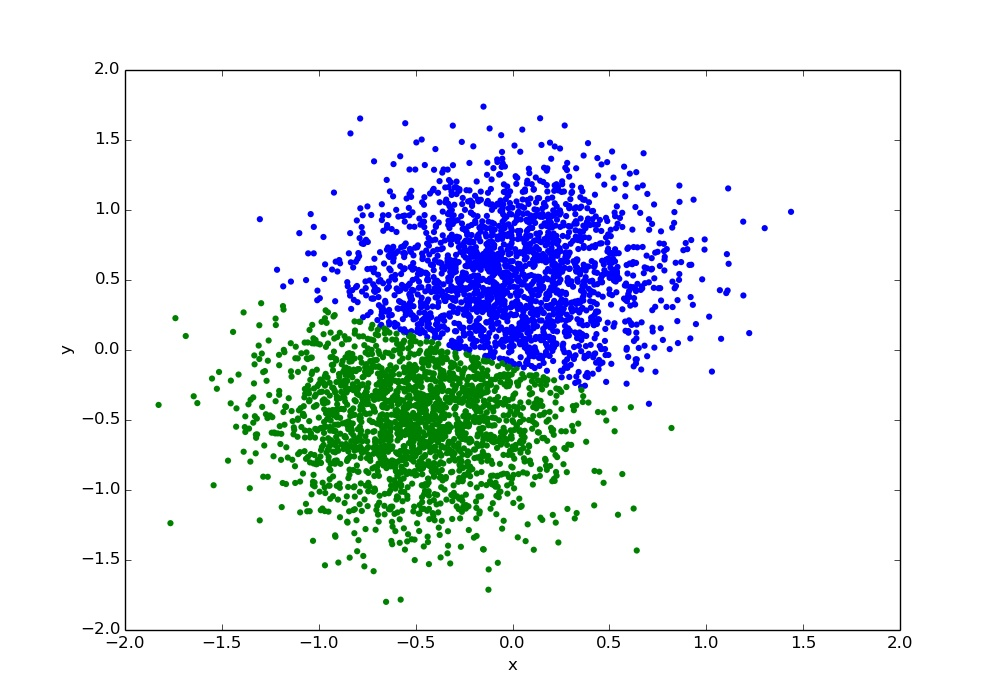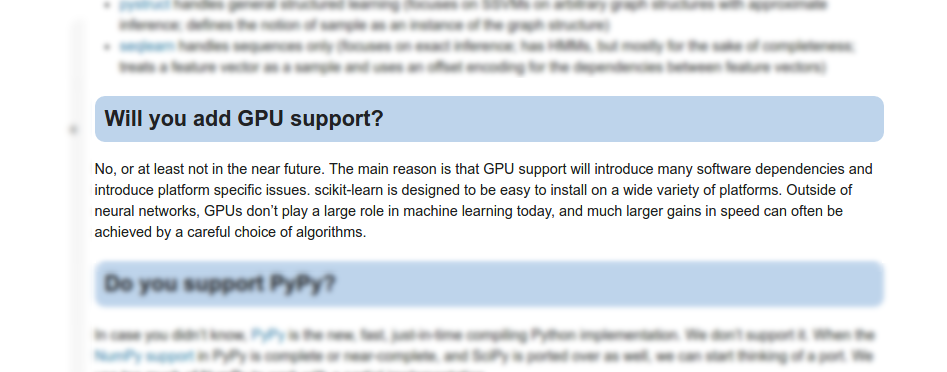# Rusty-machine ## James Lucas Note: Disclaimer: I'm a mathematician by training so things may get heavy. I'll do my best to explain but please interrupt me if I'm not making sense.
## This talk - What is machine learning? - How does rusty-machine work? - Why is rusty-machine great?

## What is rusty-machine?

Rusty-machine is a machine learning library written entirely in Rust.

It focuses on the following:

• Works out-of-the-box without relying on external dependencies.
• Simple and easy to understand API.
• Extendible and easy to configure.
## Another machine learning library? Note: - Machine learning is already in every other language, multiple times each. Are we just rewriting stuff? - Rusty-machine is more than deep learning. - Rust is a good choice: it seemed like it would be rewarding to explore.
## Machine Learning > "Field of study that gives computers the ability to learn without being explicitly programmed." - Arthur Samuel Note: We'll walk through some basic concepts in machine learning that help us to understand why rusty-machine is built as it is.

With data.

## Some examples

• Predicting rent increase
• Predicting whether an image contains a cat or a dog
• Understanding hand written digits

#### Data set might be:

rent prices and other facts about the residence.

labelled pictures of cats and dogs.

many examples of hand written digits.

## Some terminology

• Model : An object that transforms inputs into outputs based on information in data.
• Train/Fit : Teaching a model how it should transform inputs using data.
• Predict : Feeding inputs into a model to receive outputs.

To predict rent increases we may use a Linear Regression Model. We'd train the model on some rent prices and facts about the residence. Then we'd predict the rent of unlisted places.

## Why is machine learning hard?

There are many, many models to choose from.

There are many, many ways to use each model.

## Back to rusty-machine

## The foundation of rusty-machine

``````
pub trait Model<T, U> {
fn train(&mut self, inputs: &T, targets: &U);

fn predict(&self, inputs: &T) -> U;
}
``````
## An example Before we go any further we should see an example. Note: The example will show how we use these functions from the Model trait.

## K-Means

A model for clustering.## Using a K-Means Model ``` // ... Get the data samples // Create a new model with 2 clusters let mut model = KMeansClassifier::new(2); // Train the model model.train(&samples); // Predict which cluster each point belongs to let clusters : Vector<usize> = model.predict(&samples); ``` _You can run the full example in the [rusty-machine repo](https://github.com/AtheMathmo/rusty-machine/tree/master/examples)._
## Under the hood K-Means works in roughly the following way: 1. Get some initial guesses for the centroids (cluster centers) 2. Assign each point to the centroid it is closest to. 3. Update the centroids by taking the average of all points assigned to it. 4. Repeat 2 and 3 until convergence.

## K-Means Classification## Simple but complicated

The API for other models aim to be as simple as that one. However...

Machine learning is complicated.

Rusty-machine aims for ease of use.

## How does rusty-machine (try to) keep things simple?
## Using traits - A clean, simple model API - Extensibility at the user level - Reusable components within the library Note: As seen before, rusty-machine uses the `Model` trait as its foundation. This is the primary way we keep things clean and simple. We use traits to try and _hide_ as much of the machine learning complexity as possible. This is while keeping it in reach for users who need it.
## Extensibility We use traits to define parts of the models. While rusty-machine provides common defaults - users can write their own implementations and plug them in.

## Extensibility Example

#### Support Vector Machine

``````
/// A Support Vector Machine
pub struct SVM<K: Kernel> {
ker: K,
/// Some other fields
/* ... */
}
``````
``````
pub trait Kernel {
/// The kernel function.
///
/// Takes two equal length slices and returns a scalar.
fn kernel(&self, x1: &[f64], x2: &[f64]) -> f64;
}
``````

## Combining kernels

K1(x1, x2) + K2(x1, x2) = K(x1, x2)
``````
pub struct KernelSum<T, U>
where T: Kernel,
U: Kernel
{
k1: T,
k2: U,
}
``````
``````
/// Computes the sum of the two associated kernels.
impl<T, U> Kernel for KernelSum<T, U>
where T: Kernel,
U: Kernel
{
fn kernel(&self, x1: &[f64], x2: &[f64]) -> f64 {
self.k1.kernel(x1, x2) + self.k2.kernel(x1, x2)
}
}
``````

## Combining kernels

K1(x1, x2) + K2(x1, x2) = K(x1, x2)
``````
let poly_ker = kernel::Polynomial::new(...);
let hypert_ker = kernel::HyperTan::new(...);

let sum_kernel = poly_ker + hypert_ker;

let mut model = SVM::new(sum_kernel);
``````

## Reusability

We use traits to define common components, e.g. Kernels.

These components can be swapped in and out of models.

New models can easily make use of these common components.

## Reusability Example

We use Gradient Descent to minimize a cost function.

All Gradient Descent Solvers implement this trait.
``````
/// Trait for gradient descent algorithms. (Some things omitted)
pub trait OptimAlgorithm<M: Optimizable> {
/// Return the optimized parameters using gradient optimization.
fn optimize(&self, model: &M, ...) -> Vec<f64>;
}
``````

The Optimizable trait is implemented by a model which is differentiable.

## Creating a new model

Define the model.

``````
/// Cost function is: f(x) = (x-c)^2
struct XSqModel {
c: f64,
}
``````

You can think of this model as learning the value c.

## Creating a new model

Implement Optimizable for model.

``````
/// Cost function is: f(x) = (x-c)^2
struct XSqModel {
c: f64,
}

impl Optimizable for XSqModel {
/// 'params' here is 'x'
fn compute_grad(&self, params: &[f64], ...) -> Vec<f64> {
vec![2f64 * (params - self.c)]
}
}
``````

## Creating a new model

Use an OptimAlgorithm to compute the optimized parameters.

``````
/// Cost function is: f(x) = (x-c)^2
struct XSqModel {
c: f64,
}

impl Optimizable for XSqModel {
fn compute_grad(&self, params: &[f64], ...) -> Vec<f64> {
vec![2f64 * (params - self.c)]
}
}

let x_sq = XSqModel { c : 1.0 };
let x_start = vec![30.0];
let optimal = gd.optimize(&x_sq, &x_start, ...);
``````
## What can rusty-machine do? - K-Means Clustering - DBSCAN Clustering - Linear Regression - Logistic Regression - Generalized Linear Models - Neural Networks - Gaussian Process Regression - Support Vector Machines - Gaussian Mixture Models - Naive Bayes Classifiers
## Linear Algebra - [Rulinalg](https://github.com/AtheMathmo/rulinalg) Rusty-machine works without any external dependencies. Rulinalg provides linear algebra implemented entirely in Rust.

## Why Rulinalg?

Ease of use

## A quick note on error handling Rust's error handling is fantastic. ```rust impl Matrix<T> { pub fn inverse(&self) -> Result<Matrix<T>, Error> { // Fun stuff goes here } } ``` Note: Using Results to communicate that a method may fail provides more freedom whilst being more explicit. I could certainly use the error handling more frequently - especially within rusty-machine (rulinalg is pretty good).
## What does Rulinalg do? - Data structures (`Matrix`, `Vector`) - Basic operators (with in-place allocation where possible) - Decompositions (Inverse, Eigendecomp, SVD, etc.) - And more...

## Why is Rust a good choice?

• Trait system is amazing.
• Error handling is amazing.
• Performance focused code*.

* Rusty-machine needs some work, but the future looks bright!

## Why is Rust a good choice? Most importantly for me - safe control over memory. Note: Specifically with the ownership/lifetimes mechanic. We choose when a model needs ownership. When to allocate new memory for operations. These are things that are much harder to achieve in other languages as pleasant-to-use as Rust.
## When would you use rusty-machine? At the moment - experimentation, non-performance critical applications. In the future - quick, safe and powerful modeling. Note: For now it would be unwise to use this for anything serious. Except maybe if the benefits of Rust outweigh performance and accuracy. In the future, rusty-machine will try to enable rapid prototyping that can be easily extended into a finished product.
## Rust and ML in general Note: Rust is well poised to make an impact in the machine learning space. It's excellent tooling and modern design are valuable for ML - and the benefit of performance with minimal effort (once you're past wrestling with the borrow checker) is huge. Some difficulty doing 'exploratory analysis' in Rust compared to say Python. But I think in the future Rust could definitely hold it's own.

## What's next?

• Optimizing and stabilizing existing models.
• Providing optional use of BLAS/LAPACK/CUDA/etc.
## What would I like to see from Rust? - Specialization - Growth of Float/Complex generics - Continued effort from community Note: I really like the direction of the language so far and look forward to what will follow. The community is great as I'm sure most would confirm. That drive and enthusiasm will create great things.
## Summary - Machine learning (done quickly) - Rusty-machine - Rulinalg
## Contributors ||| --- | --- | --- [zackmdavis](https://github.com/zackmdavis) | [DarkDrek](https://github.com/DarkDrek) | [tafia](https://github.com/tafia) [ic](https://github.com/ic) | [rrichardson](https://github.com/rrichardson) | [vishalsodani](https://github.com/vishalsodani) [raulsi](https://github.com/raulsi) | [danlrobertson](https://github.com/danlrobertson) | [brendan-rius](https://github.com/brendan-rius) | [andrewcsmith](https://github.com/andrewcsmith) | |
## Thanks! #### Some Links - [Rusty-machine](https://github.com/AtheMathmo/rusty-machine) - [My Blog](http://athemathmo.github.io/)
## Some FAQs

## Why no GPU supportFrom Scikit-learn's FAQs.

## BLAS/LAPACK Hopefully soon!
## Integrating with other languages Nothing planned yet, but some good choices. Python is especially exciting as we gain access to lots of tooling.